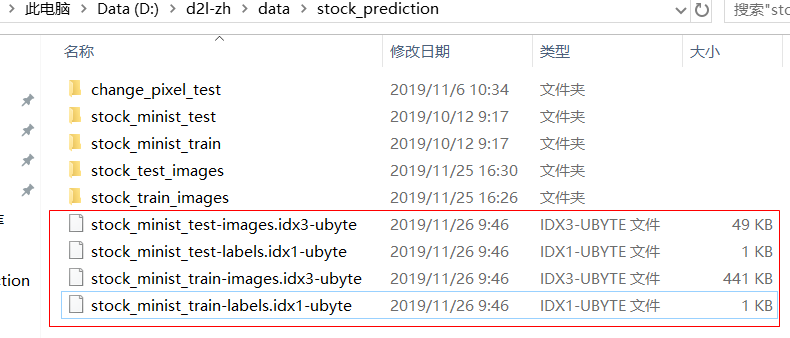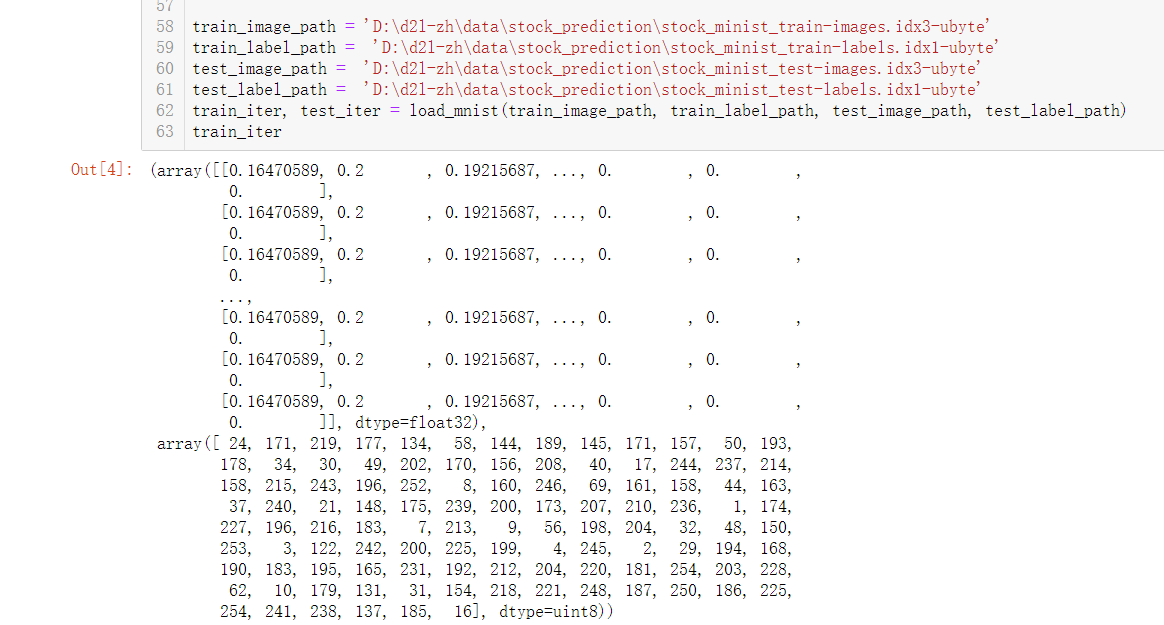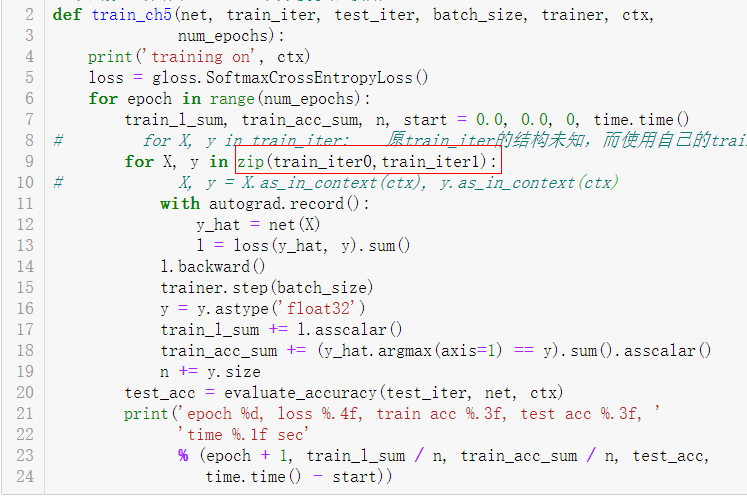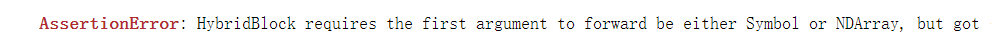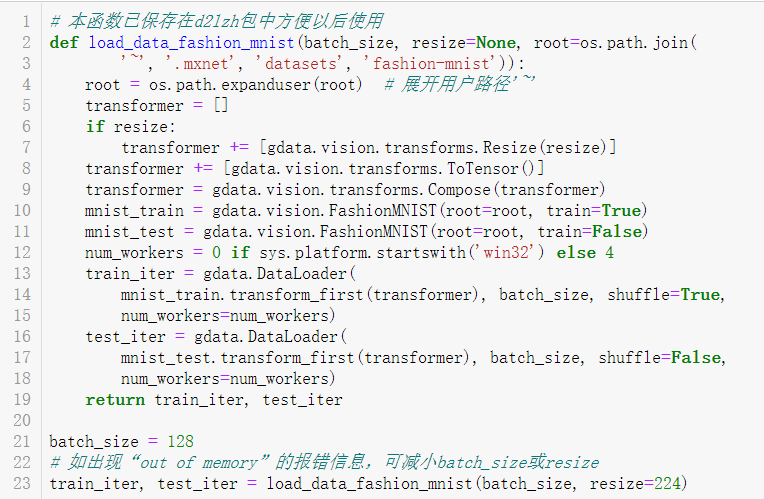``````def load_data_fashion_mnist(batch_size, resize=None, root=os.path.join(
'~', '.mxnet', 'datasets', 'fashion-mnist')):
root = os.path.expanduser(root)  # 展开用户路径'~'
transformer = []
if resize:
transformer += [gdata.vision.transforms.Resize(resize)]
transformer += [gdata.vision.transforms.ToTensor()]
transformer = gdata.vision.transforms.Compose(transformer)
mnist_train = gdata.vision.FashionMNIST(root=root, train=True)
mnist_test = gdata.vision.FashionMNIST(root=root, train=False)
num_workers = 0 if sys.platform.startswith('win32') else 4
mnist_train.transform_first(transformer), batch_size, shuffle=True,
num_workers=num_workers)
mnist_test.transform_first(transformer), batch_size, shuffle=False,
num_workers=num_workers)
return train_iter, test_iter

batch_size = 128
# 如出现“out of memory”的报错信息，可减小batch_size或resize
``````kaggle fashion-mnist.csv python的问题

1.建立了一个3个全连接层的神经网络； 2.代码如下： ``` import matplotlib as mpl import matplotlib.pyplot as plt #%matplotlib inline import numpy as np import sklearn import pandas as pd import os import sys import time import tensorflow as tf from tensorflow import keras print(tf.__version__) print(sys.version_info) for module in mpl, np, sklearn, tf, keras: print(module.__name__,module.__version__) fashion_mnist = keras.datasets.fashion_mnist (x_train_all, y_train_all), (x_test, y_test) = fashion_mnist.load_data() x_valid, x_train = x_train_all[:5000], x_train_all[5000:] y_valid, y_train = y_train_all[:5000], y_train_all[5000:] #tf.keras.models.Sequential model = keras.models.Sequential() model.add(keras.layers.Flatten(input_shape= [28,28])) model.add(keras.layers.Dense(300, activation="relu")) model.add(keras.layers.Dense(100, activation="relu")) model.add(keras.layers.Dense(10,activation="softmax")) ###sparse为最后输出为index类型，如果为one hot类型，则不需加sparse model.compile(loss = "sparse_categorical_crossentropy",optimizer = "sgd", metrics = ["accuracy"]) #model.layers #model.summary() history = model.fit(x_train, y_train, epochs=10, validation_data=(x_valid,y_valid)) ``` 3.输出结果： ``` runfile('F:/new/new world/deep learning/tensorflow/ex2/tf_keras_classification_model.py', wdir='F:/new/new world/deep learning/tensorflow/ex2') 2.0.0 sys.version_info(major=3, minor=7, micro=4, releaselevel='final', serial=0) matplotlib 3.1.1 numpy 1.16.5 sklearn 0.21.3 tensorflow 2.0.0 tensorflow_core.keras 2.2.4-tf Train on 55000 samples, validate on 5000 samples Epoch 1/10 WARNING:tensorflow:Entity <function Function._initialize_uninitialized_variables.<locals>.initialize_variables at 0x0000025EAB633798> could not be transformed and will be executed as-is. Please report this to the AutoGraph team. When filing the bug, set the verbosity to 10 (on Linux, `export AUTOGRAPH_VERBOSITY=10`) and attach the full output. Cause: WARNING: Entity <function Function._initialize_uninitialized_variables.<locals>.initialize_variables at 0x0000025EAB633798> could not be transformed and will be executed as-is. Please report this to the AutoGraph team. When filing the bug, set the verbosity to 10 (on Linux, `export AUTOGRAPH_VERBOSITY=10`) and attach the full output. Cause: 55000/55000 [==============================] - 3s 58us/sample - loss: nan - accuracy: 0.1008 - val_loss: nan - val_accuracy: 0.0914 Epoch 2/10 55000/55000 [==============================] - 3s 48us/sample - loss: nan - accuracy: 0.1008 - val_loss: nan - val_accuracy: 0.0914 Epoch 3/10 55000/55000 [==============================] - 3s 47us/sample - loss: nan - accuracy: 0.1008 - val_loss: nan - val_accuracy: 0.0914 Epoch 4/10 55000/55000 [==============================] - 3s 48us/sample - loss: nan - accuracy: 0.1008 - val_loss: nan - val_accuracy: 0.0914 Epoch 5/10 55000/55000 [==============================] - 3s 47us/sample - loss: nan - accuracy: 0.1008 - val_loss: nan - val_accuracy: 0.0914 Epoch 6/10 55000/55000 [==============================] - 3s 48us/sample - loss: nan - accuracy: 0.1008 - val_loss: nan - val_accuracy: 0.0914 Epoch 7/10 55000/55000 [==============================] - 3s 47us/sample - loss: nan - accuracy: 0.1008 - val_loss: nan - val_accuracy: 0.0914 Epoch 8/10 55000/55000 [==============================] - 3s 48us/sample - loss: nan - accuracy: 0.1008 - val_loss: nan - val_accuracy: 0.0914 Epoch 9/10 55000/55000 [==============================] - 3s 48us/sample - loss: nan - accuracy: 0.1008 - val_loss: nan - val_accuracy: 0.0914 Epoch 10/10 55000/55000 [==============================] - 3s 48us/sample - loss: nan - accuracy: 0.1008 - val_loss: nan - val_accuracy: 0.0914 ```
tensorflow CNN训练mnist数据集后识别自己写的数字效果不好

tensorflow在第一次运行Fashion MNIST会下载数据集，应该网络不好中断了报错不知咋办？
```**tensorflow在第一次运行Fashion MNIST会下载数据集，应该网络不好中断了报错不知咋办？** 代码如下： !/usr/bin/python _*_ coding: utf-8 -*- from __future__ import print_function import tensorflow as tf import matplotlib as mpl import matplotlib.pyplot as plt %matplotlib inline import numpy as np import sklearn import pandas as pd import os import sys import time from tensorflow import keras print (tf.__version__) print (sys.version_info) for module in mpl ,np, pd, sklearn, tf, keras: print (module.__name__,module.__version__) fashion_mnist = keras.datasets.fashion_mnist (x_train_all,y_train_all),(x_test,y_test) = fashion_mnist.load_data() x_valid,x_train = x_train_all[:5000],x_train_all[5000:] y_valid,y_train = y_train_all[:5000],y_train_all[5000:] print (x_valid.shape, y_valid.shape) print (x_train.shape, y_train.shape) print (x_test.shape, y_test.shape) ``` ``` 报错如下： 2.1.0 sys.version_info(major=2, minor=7, micro=12, releaselevel='final', serial=0) matplotlib 2.2.5 numpy 1.16.6 pandas 0.24.2 sklearn 0.20.4 tensorflow 2.1.0 tensorflow_core.python.keras.api._v2.keras 2.2.4-tf Traceback (most recent call last): File "/home/join/test_demo/test2.py", line 26, in <module> (x_train_all,y_train_all),(x_test,y_test) = fashion_mnist.load_data() File "/usr/local/lib/python2.7/dist-packages/tensorflow_core/python/keras/data sets/fashion_mnist.py", line 59, in load_data imgpath.read(), np.uint8, offset=16).reshape(len(y_train), 28, 28) File "/usr/lib/python2.7/gzip.py", line 261, in read self._read(readsize) File "/usr/lib/python2.7/gzip.py", line 315, in _read self._read_eof() File "/usr/lib/python2.7/gzip.py", line 354, in _read_eof hex(self.crc))) IOError: CRC check failed 0xa445bb78 != 0xe7f80d 3fL ``` ```

mnis数据集如何替换自己的数据

fashion_mnist识别准确率问题
fashion_mnist识别准确率一般为多少呢？我看好多人都是92%左右，但是我用一个网络达到了94%，想问问做过的小伙伴到底是多少？ ``` #这是我的结果示意 x_shape: (60000, 28, 28) y_shape: (60000,) epoches: 0 val_acc: 0.4991 train_acc 0.50481665 epoches: 1 val_acc: 0.6765 train_acc 0.66735 epoches: 2 val_acc: 0.755 train_acc 0.7474 epoches: 3 val_acc: 0.7846 train_acc 0.77915 epoches: 4 val_acc: 0.798 train_acc 0.7936 epoches: 5 val_acc: 0.8082 train_acc 0.80365 epoches: 6 val_acc: 0.8146 train_acc 0.8107 epoches: 7 val_acc: 0.8872 train_acc 0.8872333 epoches: 8 val_acc: 0.896 train_acc 0.89348334 epoches: 9 val_acc: 0.9007 train_acc 0.8986 epoches: 10 val_acc: 0.9055 train_acc 0.90243334 epoches: 11 val_acc: 0.909 train_acc 0.9058833 epoches: 12 val_acc: 0.9112 train_acc 0.90868336 epoches: 13 val_acc: 0.9126 train_acc 0.91108334 epoches: 14 val_acc: 0.9151 train_acc 0.9139 epoches: 15 val_acc: 0.9172 train_acc 0.91595 epoches: 16 val_acc: 0.9191 train_acc 0.91798335 epoches: 17 val_acc: 0.9204 train_acc 0.91975 epoches: 18 val_acc: 0.9217 train_acc 0.9220333 epoches: 19 val_acc: 0.9252 train_acc 0.9234667 epoches: 20 val_acc: 0.9259 train_acc 0.92515 epoches: 21 val_acc: 0.9281 train_acc 0.9266667 epoches: 22 val_acc: 0.9289 train_acc 0.92826664 epoches: 23 val_acc: 0.9301 train_acc 0.93005 epoches: 24 val_acc: 0.9315 train_acc 0.93126667 epoches: 25 val_acc: 0.9322 train_acc 0.9328 epoches: 26 val_acc: 0.9331 train_acc 0.9339667 epoches: 27 val_acc: 0.9342 train_acc 0.93523335 epoches: 28 val_acc: 0.9353 train_acc 0.93665 epoches: 29 val_acc: 0.9365 train_acc 0.9379333 epoches: 30 val_acc: 0.9369 train_acc 0.93885 epoches: 31 val_acc: 0.9387 train_acc 0.9399 epoches: 32 val_acc: 0.9395 train_acc 0.9409 epoches: 33 val_acc: 0.94 train_acc 0.9417667 epoches: 34 val_acc: 0.9403 train_acc 0.94271666 epoches: 35 val_acc: 0.9409 train_acc 0.9435167 epoches: 36 val_acc: 0.9418 train_acc 0.94443333 epoches: 37 val_acc: 0.942 train_acc 0.94515 epoches: 38 val_acc: 0.9432 train_acc 0.9460667 epoches: 39 val_acc: 0.9443 train_acc 0.9468833 epoches: 40 val_acc: 0.9445 train_acc 0.94741666 epoches: 41 val_acc: 0.9462 train_acc 0.9482 epoches: 42 val_acc: 0.947 train_acc 0.94893336 epoches: 43 val_acc: 0.9472 train_acc 0.94946665 epoches: 44 val_acc: 0.948 train_acc 0.95028335 epoches: 45 val_acc: 0.9486 train_acc 0.95095 epoches: 46 val_acc: 0.9488 train_acc 0.9515833 epoches: 47 val_acc: 0.9492 train_acc 0.95213336 epoches: 48 val_acc: 0.9495 train_acc 0.9529833 epoches: 49 val_acc: 0.9498 train_acc 0.9537 val_acc: 0.9498 ``` ``` import tensorflow as tf from tensorflow import keras import numpy as np import matplotlib.pyplot as plt def to_onehot(y,num): lables = np.zeros([num,len(y)]) for i in range(len(y)): lables[y[i],i] = 1 return lables.T # 预处理数据 mnist = keras.datasets.fashion_mnist (train_images,train_lables),(test_images,test_lables) = mnist.load_data() print('x_shape:',train_images.shape) #(60000) print('y_shape:',train_lables.shape) X_train = train_images.reshape((-1,train_images.shape*train_images.shape)) / 255.0 #X_train = tf.reshape(X_train,[-1,X_train.shape*X_train.shape]) Y_train = to_onehot(train_lables,10) X_test = test_images.reshape((-1,test_images.shape*test_images.shape)) / 255.0 Y_test = to_onehot(test_lables,10) #双隐层的神经网络 input_nodes = 784 output_nodes = 10 layer1_nodes = 100 layer2_nodes = 50 batch_size = 100 learning_rate_base = 0.8 learning_rate_decay = 0.99 regularization_rate = 0.0000001 epochs = 50 mad = 0.99 learning_rate = 0.005 # def inference(input_tensor,avg_class,w1,b1,w2,b2): # if avg_class == None: # layer1 = tf.nn.relu(tf.matmul(input_tensor,w1)+b1) # return tf.nn.softmax(tf.matmul(layer1,w2) + b2) # else: # layer1 = tf.nn.relu(tf.matmul(input_tensor,avg_class.average(w1)) + avg_class.average(b1)) # return tf.matual(layer1,avg_class.average(w2)) + avg_class.average(b2) def train(mnist): X = tf.placeholder(tf.float32,[None,input_nodes],name = "input_x") Y = tf.placeholder(tf.float32,[None,output_nodes],name = "y_true") w1 = tf.Variable(tf.truncated_normal([input_nodes,layer1_nodes],stddev=0.1)) b1 = tf.Variable(tf.constant(0.1,shape=[layer1_nodes])) w2 = tf.Variable(tf.truncated_normal([layer1_nodes,layer2_nodes],stddev=0.1)) b2 = tf.Variable(tf.constant(0.1,shape=[layer2_nodes])) w3 = tf.Variable(tf.truncated_normal([layer2_nodes,output_nodes],stddev=0.1)) b3 = tf.Variable(tf.constant(0.1,shape=[output_nodes])) layer1 = tf.nn.relu(tf.matmul(X,w1)+b1) A2 = tf.nn.relu(tf.matmul(layer1,w2)+b2) A3 = tf.nn.relu(tf.matmul(A2,w3)+b3) y_hat = tf.nn.softmax(A3) # y_hat = inference(X,None,w1,b1,w2,b2) # global_step = tf.Variable(0,trainable=False) # variable_averages = tf.train.ExponentialMovingAverage(mad,global_step) # varible_average_op = variable_averages.apply(tf.trainable_variables()) #y = inference(x,variable_averages,w1,b1,w2,b2) cross_entropy = tf.reduce_mean(tf.nn.softmax_cross_entropy_with_logits_v2(logits=A3,labels=Y)) regularizer = tf.contrib.layers.l2_regularizer(regularization_rate) regularization = regularizer(w1) + regularizer(w2) +regularizer(w3) loss = cross_entropy + regularization * regularization_rate # learning_rate = tf.train.exponential_decay(learning_rate_base,global_step,epchos,learning_rate_decay) # train_step = tf.train.GradientDescentOptimizer(learning_rate).minimize(loss,global_step=global_step) train_step = tf.train.GradientDescentOptimizer(learning_rate).minimize(loss) # with tf.control_dependencies([train_step,varible_average_op]): # train_op = tf.no_op(name="train") correct_prediction = tf.equal(tf.argmax(y_hat,1),tf.argmax(Y,1)) accuracy = tf.reduce_mean(tf.cast(correct_prediction,tf.float32)) total_loss = [] val_acc = [] total_train_acc = [] x_Xsis = [] with tf.Session() as sess: tf.global_variables_initializer().run() for i in range(epochs): # x,y = next_batch(X_train,Y_train,batch_size) batchs = int(X_train.shape / batch_size + 1) loss_e = 0. for j in range(batchs): batch_x = X_train[j*batch_size:min(X_train.shape,j*(batch_size+1)),:] batch_y = Y_train[j*batch_size:min(X_train.shape,j*(batch_size+1)),:] sess.run(train_step,feed_dict={X:batch_x,Y:batch_y}) loss_e += sess.run(loss,feed_dict={X:batch_x,Y:batch_y}) # train_step.run(feed_dict={X:x,Y:y}) validate_acc = sess.run(accuracy,feed_dict={X:X_test,Y:Y_test}) train_acc = sess.run(accuracy,feed_dict={X:X_train,Y:Y_train}) print("epoches: ",i,"val_acc: ",validate_acc,"train_acc",train_acc) total_loss.append(loss_e / batch_size) val_acc.append(validate_acc) total_train_acc.append(train_acc) x_Xsis.append(i) validate_acc = sess.run(accuracy,feed_dict={X:X_test,Y:Y_test}) print("val_acc: ",validate_acc) return (x_Xsis,total_loss,total_train_acc,val_acc) result = train((X_train,Y_train,X_test,Y_test)) def plot_acc(total_train_acc,val_acc,x): plt.figure() plt.plot(x,total_train_acc,'--',color = "red",label="train_acc") plt.plot(x,val_acc,color="green",label="val_acc") plt.xlabel("Epoches") plt.ylabel("acc") plt.legend() plt.show() ```
mnist数据集评估部分不清楚
![图片说明](https://img-ask.csdn.net/upload/201909/17/1568726719_358894.png) <br> 因为test_data是没有train过的，可以用来评估模型的accuracy等。 <br> 但是在上图中，当i%100=0时，batch是先用来输出accuracy，这时并没有train（没有把batch feed给train_step张量），那不相当于test_data么，所以可以反应模型能力？也就是说if代码块必须放在 ``` traub_step.run(feed_dict={x:batch, y_: batch, keep_prob=0.5}) ``` 之后？ <br> 那如果是这样的，那train过程中print出来的相当于没有train过的data的效果，那就根本不会出现在train中表现好，最后在test_data中表现差(除非distribution)不同？

Mnist数据集中通过灰度归一化处理反锯齿问题
The resulting images contain grey levels as a result of the anti-aliasing technique used by the normalization algorithm. the images were centered in a 28x28 image by computing the center of mass of the pixels, and translating the image so as to position this point at the center of the 28x28 field. 这是官网给的文档，请问The resulting images contain grey levels as a result of the anti-aliasing technique used by the normalization algorithm是什么操作，通过灰度归一化而达到反锯齿的效果，有没有大神解释解释，这是个什么算法？我想尽可能了解Mnist数据的来源，因为项目需要
mnist 数据库 python 加载出错 !!
RT. Anaconda python 3.4 中 加载数据出错，显示；UnicodeDecodeError: 'ascii' codec can't decode byte 0x90 in position 614: ordinal not in range(128) 代码如下： # dataset='mnist.pkl.gz' f = gzip.open(dataset, 'rb') train_set, valid_set, test_set = pickle.load(f) # 跪求四方土地，诸位大神，在此小憩片刻，帮小弟解疑答惑，感激不尽啊。
tensorboard查看mnist数据训练的问题

《奇巧淫技》系列-python！！每天早上八点自动发送天气预报邮件到QQ邮箱

11月8日，由中国信息通信研究院、中国通信标准化协会、中国互联网协会、可信区块链推进计划联合主办，科技行者协办的2019可信区块链峰会将在北京悠唐皇冠假日酒店开幕。 　　区块链技术被认为是继蒸汽机、电力、互联网之后，下一代颠覆性的核心技术。如果说蒸汽机释放了人类的生产力，电力解决了人类基本的生活需求，互联网彻底改变了信息传递的方式，区块链作为构造信任的技术有重要的价值。 　　1...

C语言魔塔游戏

Android性能优化(4)：UI渲染机制以及优化

Python爬虫爬取淘宝，京东商品信息

Java工作4年来应聘要16K最后没要,细节如下。。。

SpringBoot2.x系列教程（三十六）SpringBoot之Tomcat配置
Spring Boot默认内嵌的Tomcat为Servlet容器，关于Tomcat的所有属性都在ServerProperties配置类中。同时，也可以实现一些接口来自定义内嵌Servlet容器和内嵌Tomcat等的配置。 关于此配置，网络上有大量的资料，但都是基于SpringBoot1.5.x版本，并不适合当前最新版本。本文将带大家了解一下最新版本的使用。 ServerProperties的部分源...
Python绘图，圣诞树，花，爱心 | Turtle篇

CPU对每个程序员来说，是个既熟悉又陌生的东西？ 如果你只知道CPU是中央处理器的话，那可能对你并没有什么用，那么作为程序员的我们，必须要搞懂的就是CPU这家伙是如何运行的，尤其要搞懂它里面的寄存器是怎么一回事，因为这将让你从底层明白程序的运行机制。 随我一起，来好好认识下CPU这货吧 把CPU掰开来看 对于CPU来说，我们首先就要搞明白它是怎么回事，也就是它的内部构造，当然，CPU那么牛的一个东...

2020年全新Java学习路线图，含配套视频，学完即为中级Java程序员！！

B 站上有哪些很好的学习资源?

Web播放器解决了在手机浏览器和PC浏览器上播放音视频数据的问题，让视音频内容可以不依赖用户安装App，就能进行播放以及在社交平台进行传播。在视频业务大数据平台中，播放数据的统计分析非常重要，所以Web播放器在使用过程中，需要对其内部的数据进行收集并上报至服务端，此时，就需要对发生在其内部的一些播放行为进行事件监听。 那么Web播放器事件监听是怎么实现的呢？ 01 监听事件明细表 名...
3万字总结，Mysql优化之精髓

HTML5适合的情人节礼物有纪念日期功能